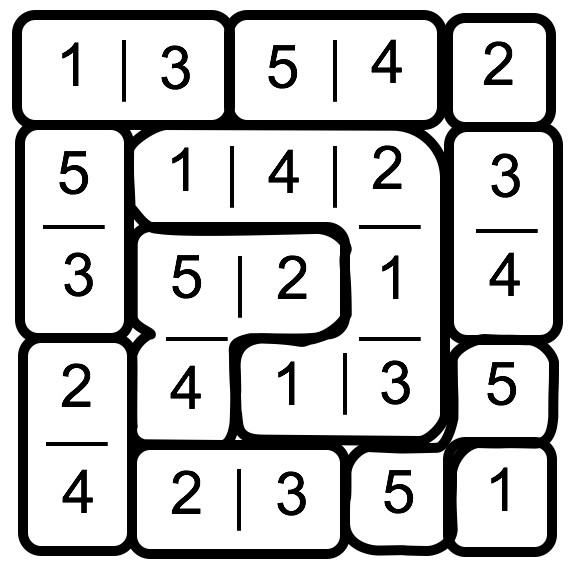## ________________

With the French Open about to begin, it’s time for another tennis puzzle.

## ________________### A Golden Set

A golden set in tennis occurs when one player wins all 24 points of a set. In the French Open, women play the best two of three sets.

What is the probability of a golden set somewhere in a specified women’s match if each point is determined by flipping a coin?

### Combining Rule

In the set of numbers below, 72 and 99 are “combined” to produce 27. Then 27 and 45 are “combined” to produce 18 and so on. The final 7 is not a misprint.

99     45     39     36     28     21
72      27     18     21      x      13     7

What is x?

## Solutions to week 141

The solution to Sudoku Variant is shown below. In Making 8 and 16, 8 = 4.7 + .8 and 16 = (.7 – .2)-4Making 8 and 16 answer explained:
8 = 4.7 + .8 = 41.5 = (22)1.5 = 23 = 8
16 = (.7 – .2)-4 = .5-4 = 1/.54 = 24 = 16

## Recent Weeks

Links to all of the puzzles and solutions are on the Complete Varsity Math page.

Come back next week for answers and more puzzles.